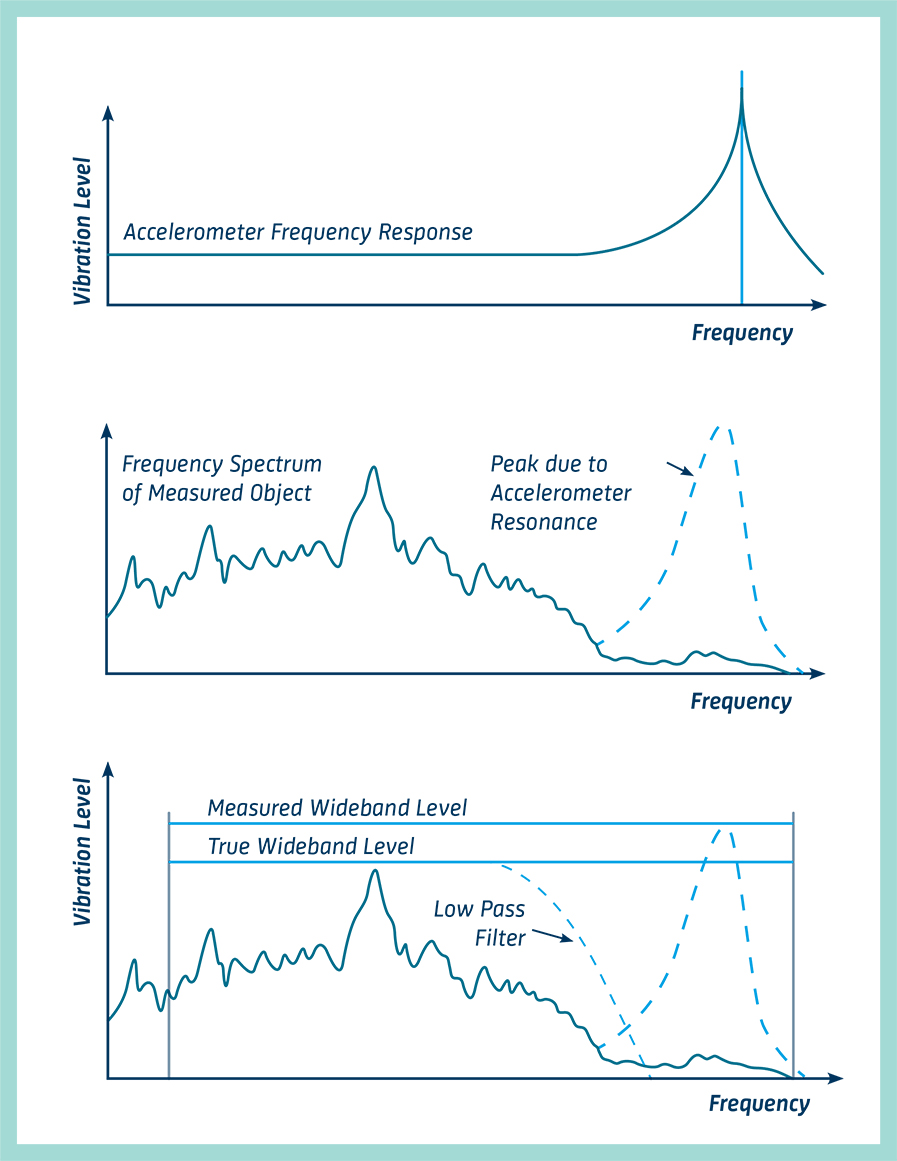# Piezoelectric Accelerometers

The Piezoelectric Accelerometer is more-or-less universally used for vibration measurements. It exhibits better all-round characteristics than any other type of vibration transducer. It has a very wide frequency and dynamic ranges with good linearity throughout the ranges. It is relatively robust and reliable so that its characteristics remain stable over a long period of time.CONTENTS

A piezoelectric accelerometer is self-generating so that it does not need a power supply. There are no moving parts to wear out, and finally, its acceleration proportional output can be integrated to give velocity and displacement proportional signals. They are capable of operation at extreme temperatures but are constrained by high output impedance requiring low-noise cables and charge amplifiers to condition the signal.

The heart of a piezoelectric accelerometer is the slice of piezoelectric material, usually an artificially polarized ferroelectric ceramic, which exhibits the unique piezoelectric effect. When it is mechanically stressed, either in tension, compression, or shear, it generates an electrical charge across its pole faces which is proportional to the applied force.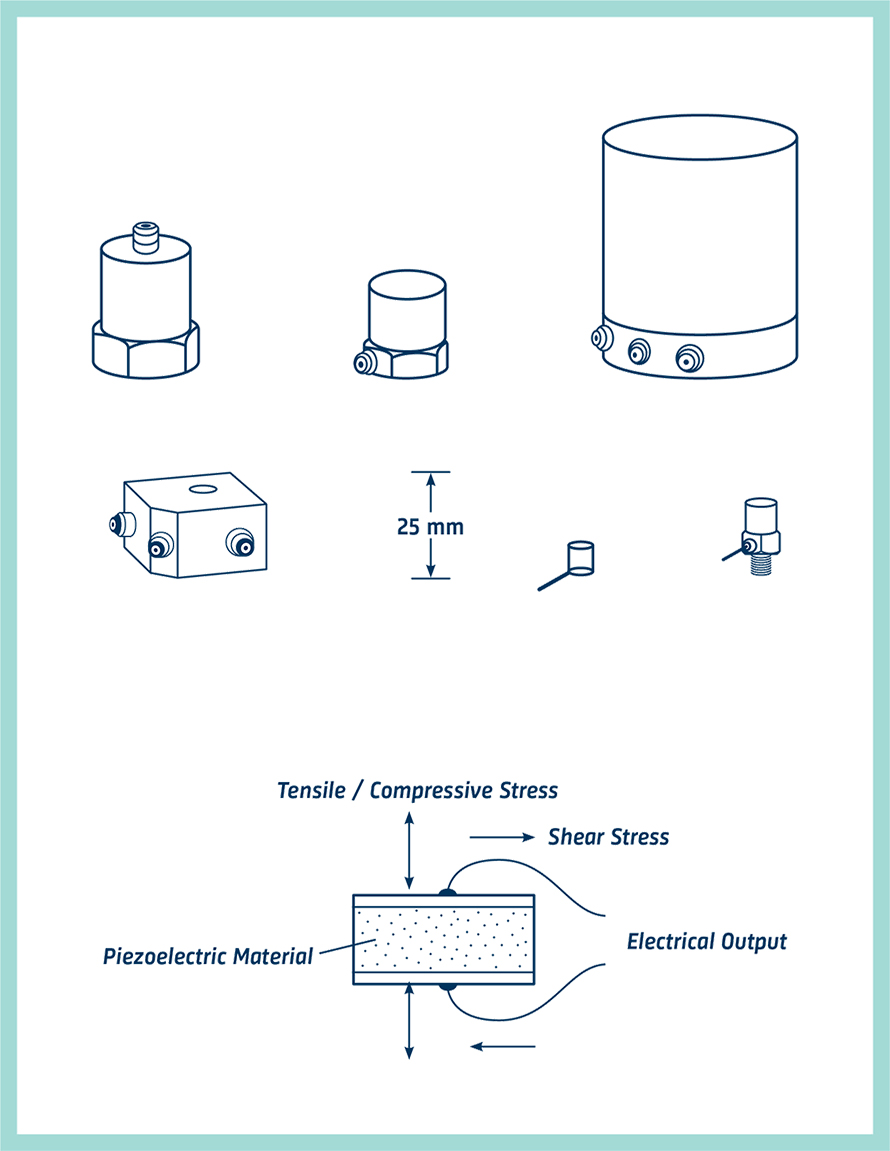## Accelerometer Designs

In practical accelerometer designs, the piezoelectric element is arranged so that when the assembly is vibrated the mass applies a force to the piezoelectric element which is proportional to the vibratory acceleration. This can be seen from the law, Force = Mass x Acceleration.

For frequencies lying well under the resonant frequency of the complete spring-mass system, the acceleration of the mass will be the same as the acceleration of the base, and the output signal magnitude will therefore be proportional to the acceleration to which the pick-up is subjected.

Two configurations are in common use:

The Compression Type where the mass exerts a compressive force on the piezoelectric element and the Shear Type where the mass exerts a shear force on the piezoelectric element.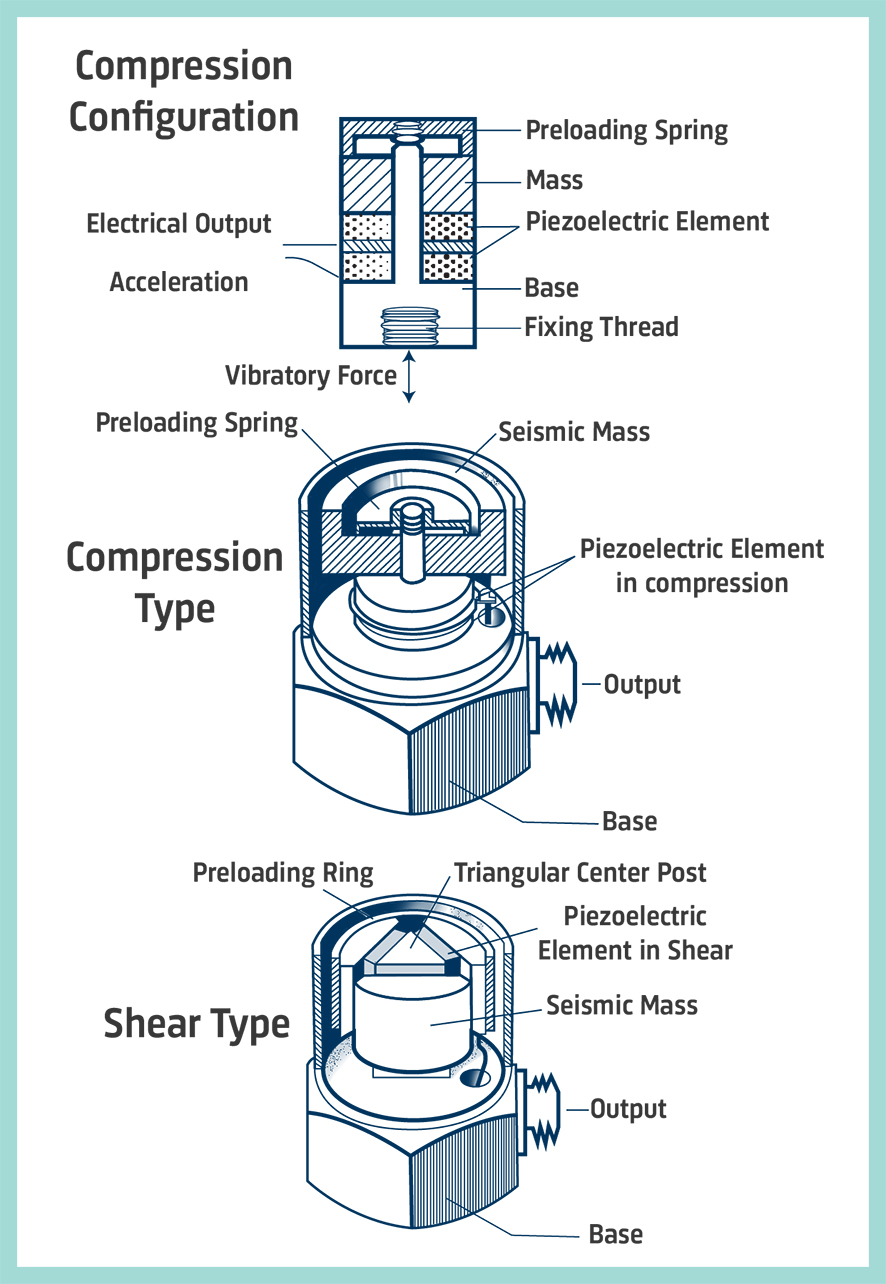## Piezoelectric Accelerometer Types

Most manufacturers have a wide range of accelerometers at first sight, maybe too many to make the choice easy.

A small group of "general purpose" types will satisfy most needs. These are available with either top or side-mounted connectors and have sensitivities in the range from 1 to 10 mV or pC per m/s2. The Brüel & Kjær Uni-Gain® types have their sensitivity normalized to a convenient "round figure" such as 1 or 10 pC/ms-2 to simplify calibration of the measuring system.

CCLD/DeltaTron® or IEPE Accelerometers

CCLD (Constant Current Line Drive) Accelerometers or IEPE (Integrated Electronics Piezo Electric) accelerometers are piezoelectric accelerometers with integral preamplifiers, which give output signals in the form of voltage modulation on the power supply line.

Brüel & Kjær's IEPE accelerometers have a high output sensitivity, high signal-to-noise ratio, and wide bandwidth make them suitable for both general purpose and high-frequency vibration measurements.

These accelerometers are high-performance instruments that have higher output sensitivity than standard piezoelectric accelerometers (without integral amplifiers). They are hermetically sealed to protect against environmental contamination, have low susceptibility to radiofrequency and electromagnetic radiation, and low impedance output due to the external constant current power source. The low-impedance output allows you to use inexpensive coaxial cables for accelerometers.

Many of the accelerometers outside the general purpose range have their characteristics slanted towards a specific application. An example; small accelerometers that are intended for high level or high-frequency measurements, and use on delicate structures, panels, etc. that weigh ca. 0,5 to 2 grams.

Other special-purpose types are optimized for simultaneous measurement in three mutually perpendicular directions; high temperatures; very low vibration levels; high-level shocks; calibration of other accelerometers by comparison; and permanent monitoring on industrial machines.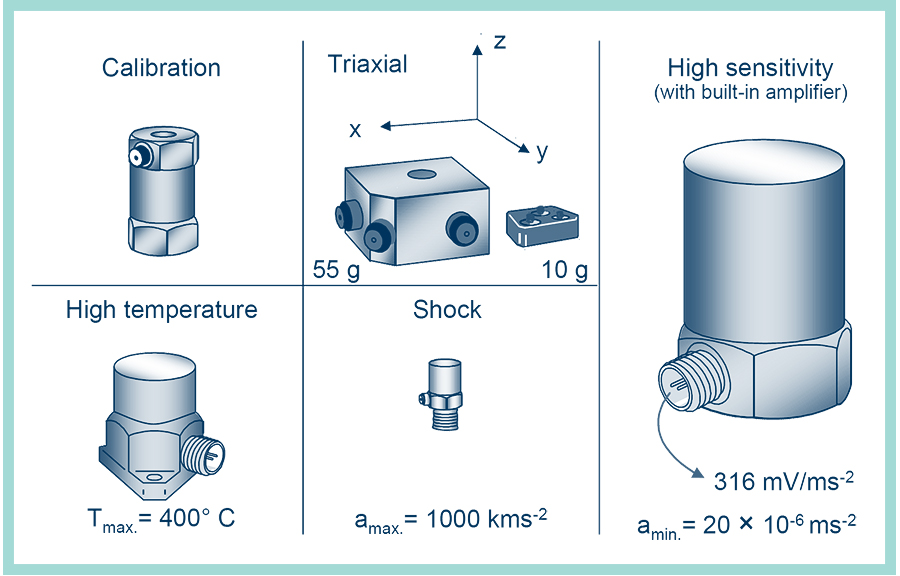## Accelerometer Sensitivity, Mass and Dynamic Range

The sensitivity is the first characteristic normally considered. Ideally, we would like a high output level, but here we must compromise because high sensitivity normally entails a relatively big piezoelectric assembly and consequently a relatively large, heavy unit.

In normal circumstances, the sensitivity is not a critical problem as modern preamplifiers are designed to accept these low-level signals. The mass of the accelerometers becomes important when measuring on light test objects. Additional mass can significantly alter the vibration levels and frequencies at the measuring point.

Generally, the accelerometer mass should be no more than one-tenth of the dynamic mass of the vibrating part onto which it is mounted. When it is wished to measure abnormally low or high acceleration levels, the dynamic range of the accelerometer should be considered.

The lower limit shown on the drawing is not normally determined directly by the accelerometer but by electrical noise from connecting cables and amplifier circuitry. This limit is normally as low as one-hundredth of an m/s2 with general-purpose instruments.

The upper limit is determined by the accelerometer's structural strength. A typical general-purpose accelerometer is linear up to 50000 to 100 000 m/s2, which is well into the range of mechanical shocks. An accelerometer especially designed for the measurement of mechanical shocks may be linear up to 1000km/s2 (100000 g).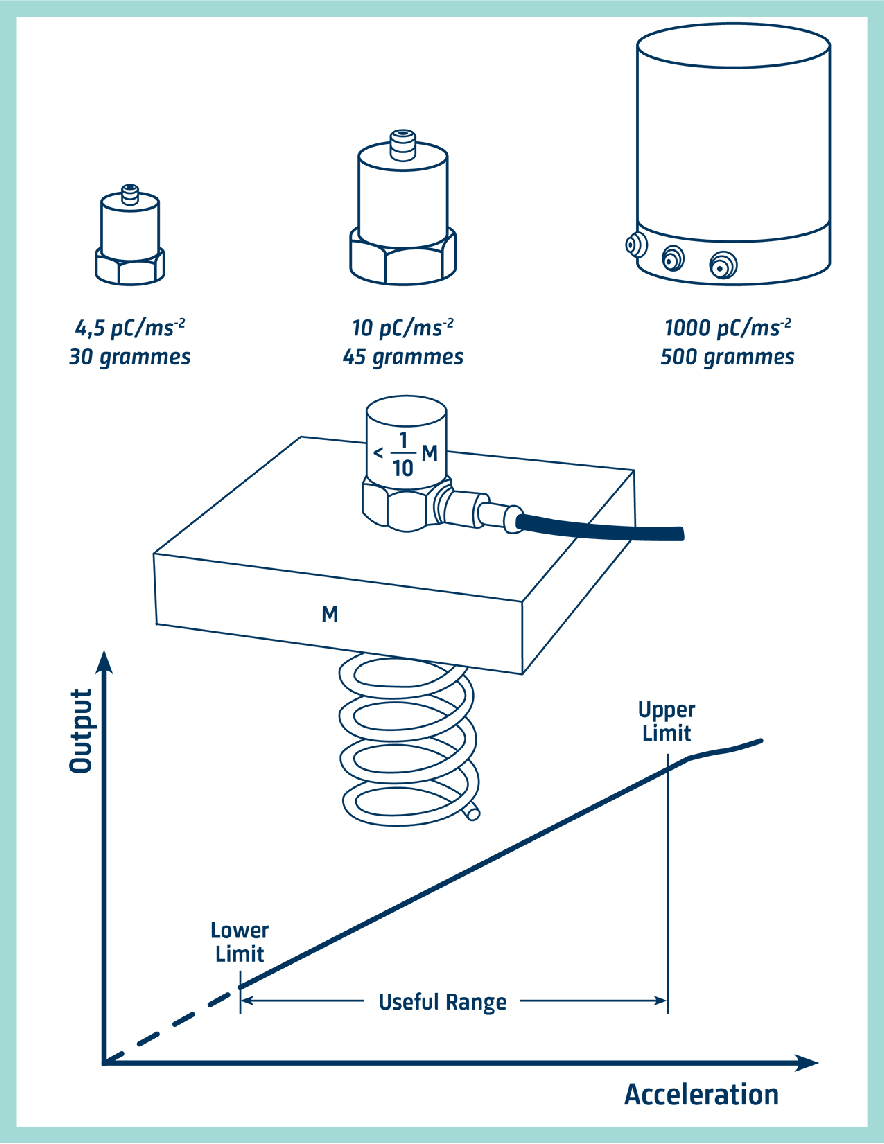## Sensor Frequency Range

Mechanical systems tend to have much of their vibration energy contained in the relatively narrow frequency range between 10 Hz to 1000 Hz, but measurements are often made up to say 10 kHz because there are often interesting vibration components at these higher frequencies. We must ensure, therefore, when selecting an accelerometer, that the frequency range of the sensor can cover the frequency range of interest.

The vibation sensor frequency range over which the accelerometer gives a true output is limited at the low-frequency end in practice, by two factors. The first is the low-frequency cut-off of the amplifier which follows it. This is not normally a problem as the limit is usually well below one Hz.

The second is the effect of ambient temperature fluctuations, to which the accelerometer is sensitive. With modern shear-type accelerometers, this effect is minimal, allowing measurements down to below 1 Hz for normal environments.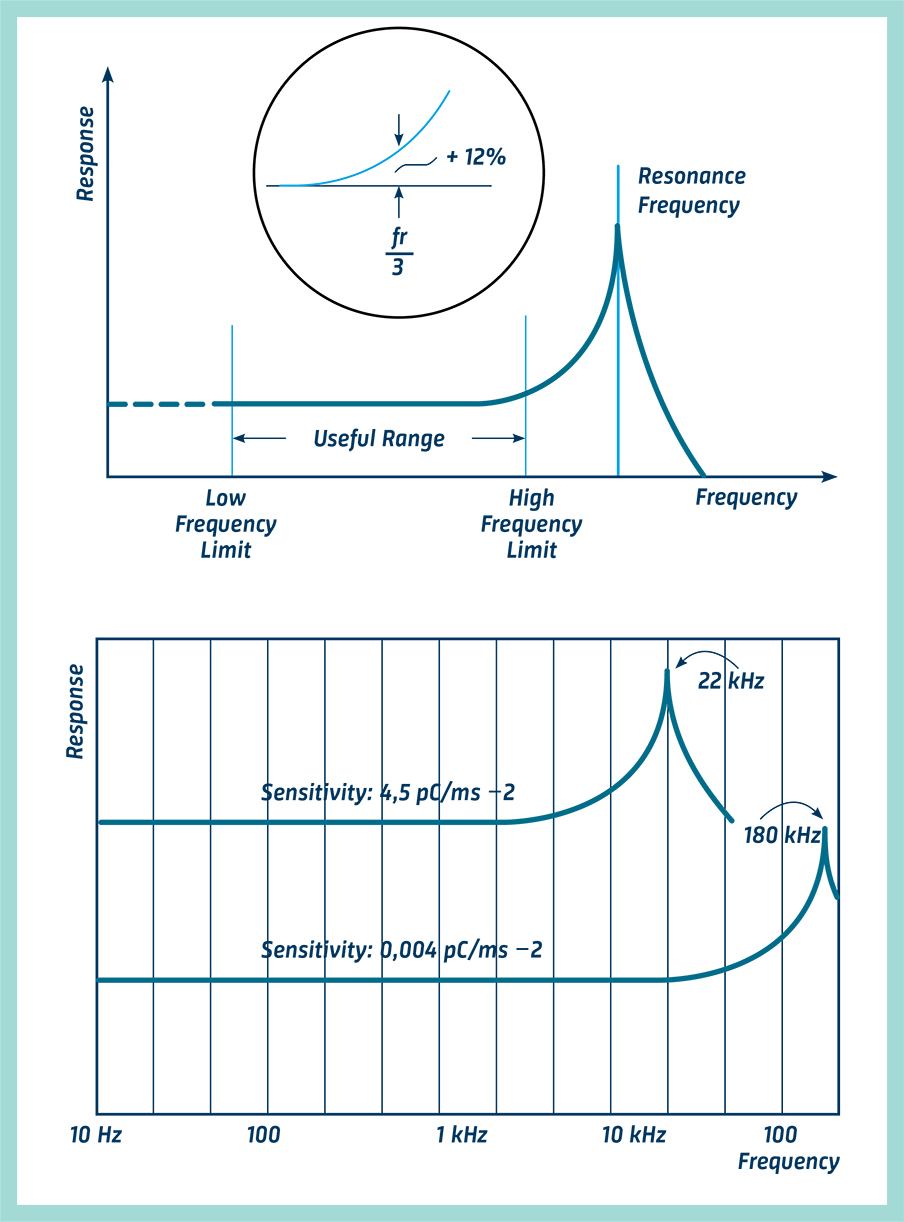The upper limit is determined by the resonant frequency of the mass-spring system of the accelerometer itself. As a rule of thumb, if we set the upper-frequency limit to one-third of the accelerometer's resonance frequency, we know that vibration measured at the upper-frequency limit of the component, will be in error by no more than + 12%.

With small accelerometers where the mass is small, the resonant frequency can be as high as 180kHz, but for the somewhat larger, higher output, general-purpose accelerometers, resonant frequencies of 20 to 30kHz are typical.

## Accelerometer Resonance Errors

As the accelerometer will typically have an increase in sensitivity at the high-frequency end due to its resonance, its output will not give a true representation of the vibration at the measuring point at these high frequencies.

When frequency analyzing a vibration signal, one may easily recognize that a high-frequency peak is due to the accelerometer resonance, and therefore ignore it. But if an overall wideband reading is taken which includes the accelerometer resonance it will give an inaccurate result if, at the same time, the vibration to be measured also has components in the region around the resonant frequency.

This problem is overcome by choosing an vibration sensor with as wide a frequency range as possible and by using a low-pass filter, which is normally included in vibration meters and preamplifiers, to cut away the undesired signal caused by the accelerometer resonance.

Where measurements are confined to low frequencies. high-frequency vibration and accelerometer resonance effects like overloads on the electronics can be removed with mechanical filters. They consist of a resilient medium, typically rubber, bonded between two mounting discs, which is mounted between the accelerometer and the mounting surface. They will typically reduce the upper-frequency limit to between 0,5 kHz to 5 kHz.

GET THE FULL HANDBOOK
MEASURING VIBRATION
BY BRÜEL & KJÆR